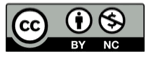# 窗户的定价规则

2019-05-10## 模型

### 玻璃的增长关系

$$C_{玻璃} ={A_{玻璃} \over A_{窗户}} = {nab \over nab } = 1$$

### 窗框的增长关系1. $n$ 扇窗户所需的窗框长度为 $2(a+b) \cdot n$
2. 一个整体的外框： $2(a+nb)$

\begin{align} L_{型材} &= 2(a+b) \cdot n+2(a+bn) \\ &= 2an+2bn+2a+2bn \\ &= 2(a+2b)n+2a\end{align}

\begin{align} C_{型材} &={L_{型材} \over A_{窗户}} \\ &= {{2(a+2b)n+2a} \over {nab}} \\&= {{2(a+2b) \over ab}+{2 \over {nb}}}\\ &={{2 \over b}+{4 \over a}+{2 \over nb}} \end{align}

### 窗框的成本趋势

\begin{align}\rho(n) &={{2 \over nb} \over {{2 \over b}+{4 \over a}+{2 \over nb}}} \\ &={{2a \over nab} \over {{2an+4bn+2a}\over{nab}}}\\ &={{a}\over {(a+2b)n+a}} \end{align}

\begin{align}\rho(n) &={{a}\over {(a+a)n+a}}\\&={{1}\over {2n+1}}\end{align}（完）

## 精彩评论